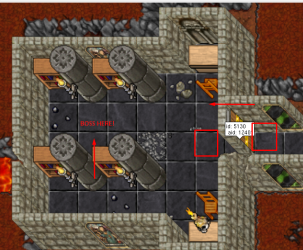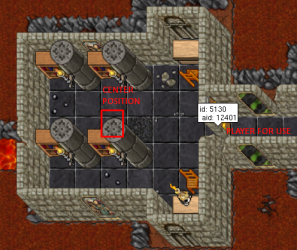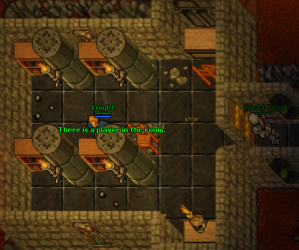# TFS 1.X+1 Players for a Room (Boss, Check Area, Time) - Actions

#### Fabi Marzan

##### Member
Greetings as always again here with my problems.

I've been trying to figure out how to make it so that when a player uses the door, the other players will have to wait a certain time to use it again, another thing is how to make it so that if a player is in an area, the other players can't use the door... that comes out as "There is a player inside".

My idea is that when the player uses the door, a boss appears, so it is only for one player.

On the area to check if there is a player I have used the function:

Lua:
``````local cfg = {
centrePosition = Position(1005, 1008, 6),
xRange = 5, -- smallest size you can use is 1. do not put 0, or it's range defaults to 12.
yRange = 5, -- (range 1 is an 'exori' 3 by 3 tiles. range 2 is 5 by 5 tiles)
multifloor = false, -- if you need to check all floors, not only Z floor from centrePosition
onlyPlayers = true,
}

local spectators = Game.getSpectators(cfg.centrePosition, cfg.multifloor, cfg.onlyPlayers, cfg.xRange, cfg.xRange, cfg.yRange, cfg.yRange)
for i = 1, #spectators do
local player = spectators[i]
if i == #spectators then
end
end``````

But I don't know if it validates that function.

Here's an image for context:This is the scripts that I'm putting together. If the player does not have the storage (91154), it cannot pass then when obtaining the storage (91154), when it tries again to open the door it passes to (91155),
which is where you can't go back.

Code:
``````local action = Action()
function action.onUse(cid, player, item, fromPosition, target, toPosition, isHotkey)
local player = Player(cid)
if not player then
return true
end
if getCreatureStorage(cid, 91155) == 1 then
player:getPosition():sendMagicEffect(3)
player:sendTextMessage(25, "There is no way back.")   ------- PLAYER NOT BACK, So as not to spam (Storage - 91154)
return true
end
if getCreatureStorage(cid, 91154) == 1 then
player:getPosition():sendMagicEffect(11)
player:teleportTo(Position(10154, 10200, 7))
player:setDirection(DIRECTION_WEST)
player:sendTextMessage(25, "Be careful in 5 seconds the boss Drobura will appear...")
player:say("Be careful in 5 seconds the boss Drobura will appear...")
player:setStorageValue(91155, 1)
else
player:sendCancelMessage("You don't have the necessary items to pass. [Not Permission].")
return true
end
end``````

I know, my way of making scripts is very basicI only need all the others, and it would be 100% scripts, which would be the boss, a room check so that no more players can enter,
a timer that only one player can enter and to use the door again they have to wait a certain time.

I would like that if the player does not kill the boss in 15min for example, he teleports and the boss disappears. I say this for troll people.

Solution
So first things first..
This line is weird.
Lua:
``onUse(cid, player, item, fromPosition, target, toPosition, isHotkey)``
You can technically rename these.. but there's no point to do so.
Replacing it with the standard.
Lua:
``onUse(player, item, fromPosition, target, toPosition, isHotkey)``

With that change, we can remove this entire section
Lua:
``````    local player = Player(cid)
if not player then
return true
end``````

The reason it's sending the player in the room the message, is because you replaced 'player'
Lua:
``````local spectators = Game.getSpectators(cfg.centrePosition, cfg.multifloor, cfg.onlyPlayers, cfg.xRange, cfg.xRange, cfg.yRange, cfg.yRange)
for i = 1, #spectators do
local player = spectators[i] --...``````
In your loop you are checking for all players found in the area..
Lua:
``````for i = 1, #spectators do
local player = spectators[i]
if i == #spectators then
end
end``````
Modifying your loop very slightly.. will give you a check for players in the room.
Lua:
``````for i = 1, #spectators do
local player = spectators[i]
if player then
-- player found in room. Now do what?
return true -- this would stop the door from functioning, by stopping the script here.
end
end``````

Towards that end, note that 5 by 5 tiles reaches 5 tiles outwards from the centre position, meaning it's an 11 by 11 tile squared area.
Just be mindful that the players using the door are not in this area you have chosen.

@Xikini I still don't understand very well, what I did was this:the message goes out to the one inside.Forgive the scripts that are disorganized, it is only for testing the scripts...
in centreposition: place the pos of the room area does not reach the door.

Code:
``````local cfg = {
centrePosition = Position(10151, 10200, 7),
xRange = 3, -- smallest size you can use is 1. do not put 0, or it's range defaults to 12.
yRange = 3, -- (range 1 is an 'exori' 3 by 3 tiles. range 2 is 5 by 5 tiles)
multifloor = false, -- if you need to check all floors, not only Z floor from centrePosition
onlyPlayers = true,
}
local action = Action()
function action.onUse(cid, player, item, fromPosition, target, toPosition, isHotkey)
local player = Player(cid)
if not player then
return true
end
if getCreatureStorage(cid, 91155) == 1 then
player:getPosition():sendMagicEffect(3)
player:sendTextMessage(25, "There is no way back.")
return true
end
if getCreatureStorage(cid, 91154) == 1 then
local spectators = Game.getSpectators(cfg.centrePosition, cfg.multifloor, cfg.onlyPlayers, cfg.xRange, cfg.xRange, cfg.yRange, cfg.yRange)
for i = 1, #spectators do
local player = spectators[i]
if player then
player:sendTextMessage(25, "There is a player in the room.") -- -- player found in room.
return true -- this would stop the door from functioning, by stopping the script here.
end
end
player:getPosition():sendMagicEffect(11)
player:teleportTo(Position(10154, 10200, 7))
player:setDirection(DIRECTION_WEST)
player:sendTextMessage(25, "Be careful in 5 seconds the boss Drobura will appear...")
player:say("Be careful in 5 seconds the boss Drobura will appear...")
else
player:sendCancelMessage("You don't have the necessary items to pass. [Not Permission].")
return true
end
end

action:aid(12401)
action:register()``````

So first things first..
This line is weird.
Lua:
``onUse(cid, player, item, fromPosition, target, toPosition, isHotkey)``
You can technically rename these.. but there's no point to do so.
Replacing it with the standard.
Lua:
``onUse(player, item, fromPosition, target, toPosition, isHotkey)``

With that change, we can remove this entire section
Lua:
``````    local player = Player(cid)
if not player then
return true
end``````

The reason it's sending the player in the room the message, is because you replaced 'player'
Lua:
``````local spectators = Game.getSpectators(cfg.centrePosition, cfg.multifloor, cfg.onlyPlayers, cfg.xRange, cfg.xRange, cfg.yRange, cfg.yRange)
for i = 1, #spectators do
local player = spectators[i] -- replacing it here, with the spectator found in the room.
if player then
player:sendTextMessage(25, "There is a player in the room.")
return true
end
end``````
So to fix that, we just need to name it something else. `_player` is a popular choice.

While we're in here, we'll replace the couple of functions that are using compatability file..

Example:
Lua:
``````getCreatureStorage(cid, 91155) -- compat version
player:getStorageValue(91155) -- normal``````

and we'll get rid of the config table, because none of the values are being used multiple times...
And use a faster/better version then the looping spectators function.. because we don't really care about the person/people in the room

Lua:
``````local action = Action()

function action.onUse(player, item, fromPosition, target, toPosition, isHotkey)
if player:getStorageValue(91155) == 1 then
player:getPosition():sendMagicEffect(3)
player:sendTextMessage(25, "There is no way back.")
return true
end
if player:getStorageValue(91154) ~= 1 then
player:sendCancelMessage("You don't have the necessary items to pass. [Not Permission].")
return true
end

-- This is an alternative to the above looping through the spectators option, which is cleaner.

local spectators = Game.getSpectators(Position(10151, 10200, 7), false, true, 3, 3, 3, 3)
if spectators and #spectators > 0 then
player:sendTextMessage(25, "There is a player in the room.") -- tell person using the door that player is in room
return true -- stop looping through spectators, and block door access.
end

player:getPosition():sendMagicEffect(11)
player:teleportTo(Position(10154, 10200, 7))
player:setDirection(DIRECTION_WEST)
player:sendTextMessage(25, "Be careful in 5 seconds the boss Drobura will appear...")
player:say("Be careful in 5 seconds the boss Drobura will appear...")
return true
end

action:aid(12401)
action:register()``````

@Xikini Oohh ok I'm starting to understand, another thing I don't understand is the symbols that are placed after the storages.
These:

Lua:
``````~=
==
>=``````

Which is the way to use each symbol depending on the scripts.

Ex:
Code:
``if player:getStorageValue(88914) >= 1 then``

Because testing, I have changed the one below in ==
Code:
`````` if player:getStorageValue(91153) == 1 then
player:getPosition():sendMagicEffect(3)
player:sendCancelMessage("You don't have the necessary items to pass. [Not Permission].")
return true
end
if player:getStorageValue(91154) == 1 then
player:getPosition():sendMagicEffect(11)
player:teleportTo(Position(10154, 10200, 7))
player:setDirection(DIRECTION_WEST)
player:sendTextMessage(25, "Be careful in 5 seconds the boss Drobura will appear...")
player:say("Be careful in 5 seconds the boss Drobura will appear...")
player:setStorageValue(91154, 1)
return true
end``````

So, there I realized that it ignores the spectactors function.

@Xikini Oohh ok I'm starting to understand, another thing I don't understand is the symbols that are placed after the storages.
These:

Lua:
``````~=
==
>=``````

Which is the way to use each symbol depending on the scripts.

Ex:
Code:
``if player:getStorageValue(88914) >= 1 then``

Because testing, I have changed the one below in ==
Code:
`````` if player:getStorageValue(91153) == 1 then
player:getPosition():sendMagicEffect(3)
player:sendCancelMessage("You don't have the necessary items to pass. [Not Permission].")
return true
end
if player:getStorageValue(91154) == 1 then
player:getPosition():sendMagicEffect(11)
player:teleportTo(Position(10154, 10200, 7))
player:setDirection(DIRECTION_WEST)
player:sendTextMessage(25, "Be careful in 5 seconds the boss Drobura will appear...")
player:say("Be careful in 5 seconds the boss Drobura will appear...")
player:setStorageValue(91154, 1)
return true
end``````

So, there I realized that it ignores the spectactors function.

They are Relational Operators.

The goal is to return a true or false statement.
If the statement is true, go into the if statement.
If false, skip past and continue on the code.

Lua:
``````-- Do these two values equal one another?
if 3 == 5 then -- false
if 3 == 3 then -- true

-- Do these two values NOT equal one another?
if 3 ~= 5 then -- true
if 3 ~= 3 then -- false

-- Is this value greater then this other value?
if 3 > 5 then -- false
if 3 > 3 then -- false
if 3 > 0 then -- true

-- Is this value less then this other value?
if 3 < 5 then -- true
if 3 < 3 then -- false
if 3 < 0 then -- false

-- Is this value greater then or equal to this other value?
if 3 >= 5 then -- false
if 3 >= 3 then -- true
if 3 >= 0 then -- true

-- Is this value less then or equal to this other value?
if 3 <= 5 then -- true
if 3 <= 3 then -- true
if 3 <= 0 then -- false``````

They are Relational Operators.

The goal is to return a true or false statement.
If the statement is true, go into the if statement.
If false, skip past and continue on the code.

Lua:
``````-- Do these two values equal one another?
if 3 == 5 then -- false
if 3 == 3 then -- true

-- Do these two values NOT equal one another?
if 3 ~= 5 then -- true
if 3 ~= 3 then -- false

-- Is this value greater then this other value?
if 3 > 5 then -- false
if 3 > 3 then -- false
if 3 > 0 then -- true

-- Is this value less then this other value?
if 3 < 5 then -- true
if 3 < 3 then -- false
if 3 < 0 then -- false

-- Is this value greater then or equal to this other value?
if 3 >= 5 then -- false
if 3 >= 3 then -- true
if 3 >= 0 then -- true

-- Is this value less then or equal to this other value?
if 3 <= 5 then -- true
if 3 <= 3 then -- true
if 3 <= 0 then -- false``````
Ah, it's like math classes "inequities", thanks for the doubts, and my scripts worked as I wanted, I just need to add everything else, the boss and a time limit in the room and I'd be ready, I'll be checking the other posts. Thank you.

T
TFS 1.X+ boss room check
Replies
7
Views
495
Tibia Demon
T
Replies
5
Views
479
T
Replies
0
Views
176
Tibia Demon
T
Replies
0
Views
206
Replies
1
Views
209
T
TFS 1.X+ on step in area
Replies
4
Views
373
Tibia Demon
T